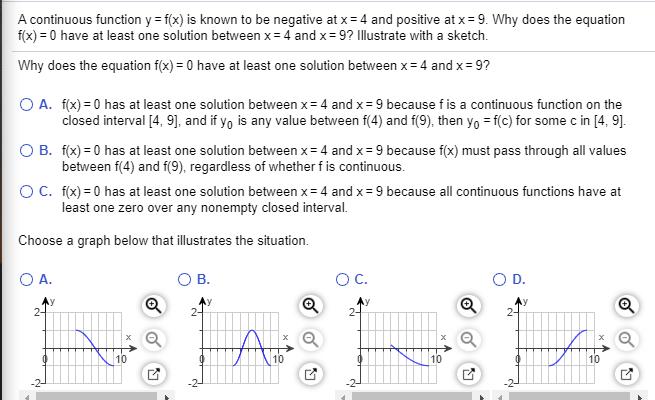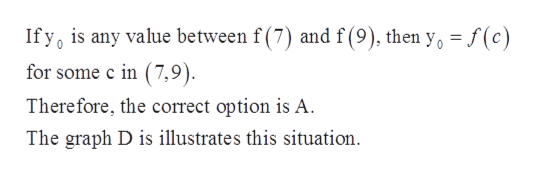# A continuous function y = f(x) is known to be negative at x= 4 and positive at x = 9. Why does the equationf(x) = 0 have at least one solution between x= 4 and x= 9? Illustrate with a sketch.Why does the equation f(x) = 0 have at least one solution between x= 4 and x= 9?O A. f(x) = 0 has at least one solution between x= 4 and x= 9 because f is a continuous function on theclosed interval [4, 9], and if y, is any value between f(4) and f(9), then yo = f(c) for some c in [4, 9].O B. f(x) = 0 has at least one solution between x= 4 and x= 9 because f(x) must pass through all valuesbetween f(4) and f(9), regardless of whether f is continuous.OC. f(x) = 0 has at least one solution between x= 4 and x= 9 because all continuous functions have atleast one zero over any nonempty closed interval.Choose a graph below that illustrates the situation.O A.OC.B.D.Ay2-Ay2-2-2-101010

Question
77 views

*show work*help_outlineImage TranscriptioncloseA continuous function y = f(x) is known to be negative at x= 4 and positive at x = 9. Why does the equation f(x) = 0 have at least one solution between x= 4 and x= 9? Illustrate with a sketch. Why does the equation f(x) = 0 have at least one solution between x= 4 and x= 9? O A. f(x) = 0 has at least one solution between x= 4 and x= 9 because f is a continuous function on the closed interval [4, 9], and if y, is any value between f(4) and f(9), then yo = f(c) for some c in [4, 9]. O B. f(x) = 0 has at least one solution between x= 4 and x= 9 because f(x) must pass through all values between f(4) and f(9), regardless of whether f is continuous. OC. f(x) = 0 has at least one solution between x= 4 and x= 9 because all continuous functions have at least one zero over any nonempty closed interval. Choose a graph below that illustrates the situation. O A. OC. B. D. Ay 2- Ay 2- 2- 2- 10 10 10 fullscreen
check_circle

Step 1

Given:

A continuous function y = f (x) is known to be negative at x = 4 and positive at x = 9.

Step 2

Concept used:

Intermediate Value Theorem said that:

If f (x) is a continuous function on [a, b], then for every k between f (a) and f (b), there exists a value c belongs to (a, b) such that f (c) = k.

Step 3

By Intermediate Value Theorem, f (x) = 0 has at least one solution between...help_outlineImage TranscriptioncloseIf y, is any value between f (7) and f(9), then y, = f (c) %3D for some c in (7,9). Therefore, the correct option is A. The graph D is illustrates this situation. fullscreen

### Want to see the full answer?

See Solution

#### Want to see this answer and more?

Solutions are written by subject experts who are available 24/7. Questions are typically answered within 1 hour.*

See Solution
*Response times may vary by subject and question.
Tagged in
MathCalculus

### Other